Courses

# Particle in a Box Problem - The Schrodinger Equation, Quantum Mechanics, CSIR-NET Physical Sciences Physics Notes | EduRev

## Physics for IIT JAM, UGC - NET, CSIR NET

Created by: Akhilesh Thakur

## Physics : Particle in a Box Problem - The Schrodinger Equation, Quantum Mechanics, CSIR-NET Physical Sciences Physics Notes | EduRev

The document Particle in a Box Problem - The Schrodinger Equation, Quantum Mechanics, CSIR-NET Physical Sciences Physics Notes | EduRev is a part of the Physics Course Physics for IIT JAM, UGC - NET, CSIR NET.
All you need of Physics at this link: Physics

We will now look at the solutions of a particle of mass m con ned to move along the x-axis between 0 to L. This is achieved by making the potential 0 between x = 0 and x = L and V = ∞ for x < 0 and x > L (see Figure 1). In quantum mechanics this model is referred to as particle in a box (PIB) of length L.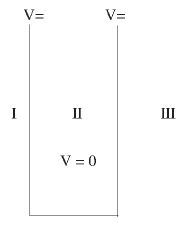Figure 1: The one-dimensional in nite potential well of length L. It is divided into three regions, with regions I and III having V = ∞ and region II having V = 0

Before we set up and solve the Schrodinger equation let us apply de Broglie's approach to this problem. de Broglie associates a wave with every material particle traveling with momentum p. The wavelength of the wave is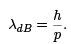The classical analog of a particle in a box is a string that is xed at both ends. When such a string is plucked, we know that the amplitude of the oscillations at the xed ends is zero. In other words, an integer number of half-wavelengths must t in the length of the box. Applying this idea to the present case, we nd that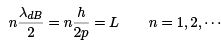Solving for p we get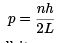The particle feels no potential energy so all its energy is in the form of kinetic energy. As a result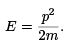Substituting for p, we get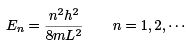Let us now proceed to analyze this problem quantum mechanically. The Schrodinger equation is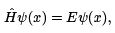(1)

where V (x) = ∞ in regions I and III and V (x) = 0 in region II. The results are in no way a effected if in region II the potential is V because it only has the effect of altering the zero of energy and so without of generality we will assume that V = 0 within the box.
In regions I and III the wave function is identically zero since the potential is in nite so we will only have to consider the solution within the box.
We are looking for the solutions to the equation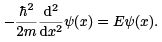(2)
We rewrite this differential equation in the form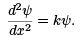(3)

where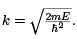The general solution to this differential equation is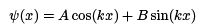where A and B are constants. (Verify that this indeed is the solution by substituting it into the differential equation.) The boundary conditions that the wave function should satisfy are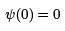and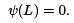The first of these conditions implies that A = 0. The second condition yields B sin(kL) = 0 which implies that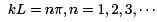or in other words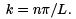. Because E is related to k we hence obtain that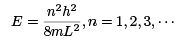and the wave function has the form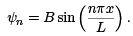You will notice that the application of the boundary conditions naturally leads to quantization. In addition, observe that we do not consider n = 0 as a possibility because that would imply that the wave function is identically zero for all x or in other words there is no particle!
The constant B is yet to be determined. The normalization condition on the wave function yields B .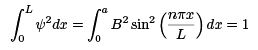from which we determine that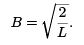Interestingly, the energies obtained by solving the Schrodinger equation are identical to that obtained from a qualitative analysis using the de Broglie principle. Observe that the lowest state has an energy of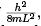which is different from zero. This is called the zero point energy. It implies that even when the system is in the ground state it is undergoing ceaseless motion. This zero point energy is a result of the uncertainty principle.
What is the zero point energy predicted by the uncertainty principle? The particle is con ned to a box of length L, which means that the uncertainty in the position is L - the particle has to be somewhere in the box. The minimum uncertainty in the momentum from the Heisenberg relation is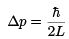The momentum is at least of the order of the uncertainty in the momentum, implying that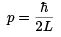or in other words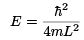a result that agrees with the quantitative result derived in the last paragraph.
Having determined the wave function for this problem let us put it to some use. One of the things that we are often interested in is the position of the particle if it is present in a particular state. Notice that the states that we solved for are energy eigen states. Moreover, they are not eigen states position. That is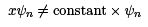In cases where the wave function is not an eigen function of the operator corresponding to the observable, we will be concerned with the statistical mean of the measured values, averaged over a large number of measurements, the expectation value. The expectation value is de ned of an operator is de ned as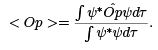The expectation value of the position for a particle in the n-th state of a particle in a box would hence be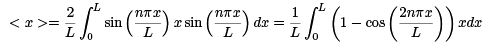Because the wave functions are normalized the denominator is unity. You can show, either by symmetry arguments or by explicit integration, that the result is L=2, which agrees with the classical result.
This process may be performed for any other observable. For example, the average momentum or the expectation value of the momentum of a particle in the n-th state of the box. The only difference in the procedure to determine the expectation value of position is to replace the position operator with the momentum operator. That is, multiplication by x is replaced with the momentum operator,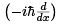The average momentum is then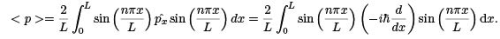By symmetry arguments, we fi nd that the average momentum is zero. This agrees with the classical result - the particle has equal probability of traveling in either the +x or x direction.

159 docs

,

,

,

,

,

,

,

,

,

,

,

,

,

,

,

,

,

,

,

,

,

,

,

,

,

,

,

;